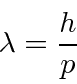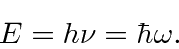# The Solution: Probability Amplitudes

For EM waves, the intensity, and hence the probability to find a photon, is proportional to the square of the fields. The fields obey the wave equation. The fields from two slits can add constructively or destructively giving interference patterns. Theandfields are 90 degrees out of phase and both contribute to the intensity.

We will use the same ideas for electrons, although the details of the field will vary a bit because electrons and photons are somewhat different kinds of particles. For both particles the wavelength is given byand the frequency byWe will use a complex probability amplitudefor the electron. The real and imaginary parts are out of phase like the EM fields. The traveling wave with momentumand energythen isThe probability to find an electron is equal to the absolute square of the complex probability amplitude.(We will overcome the problem that this probability is 1 everywhere for our simple wavefunction.)

We have just put in most of the physics of Quantum Mechanics. Much of what we do for the rest of the course will be deduced from the paragraph above. Our input came from deBroglie and Plank, with support from experiments.

Lets summarize the physics input again.

• Free particles are represented by complex wave functions with a relationship between their particle properties - energy and momentum, and their wave properties - frequency and wavelength given by Plank and deBroglie.
• The absolute square of the wavefunction gives the probability distribution function. Quantum Mechanics only tells us the probability.
• We can make superpositions of our free particle wave functions to make states that do not have definite momentum. We will find that any state can be made from the superposition of free particle states with different momentum.

We now have a wave-particle duality for all the particles, however, physics now only tells us the probability for some quantum events to occur. We have lost the complete predictive power of classical physics.

Gasiorowicz Chapter 1

Rohlf Chapter 5

Griffiths 1.2, 1.3

Cohen-Tannoudji et al. Chapter

Subsections
Jim Branson 2013-04-22